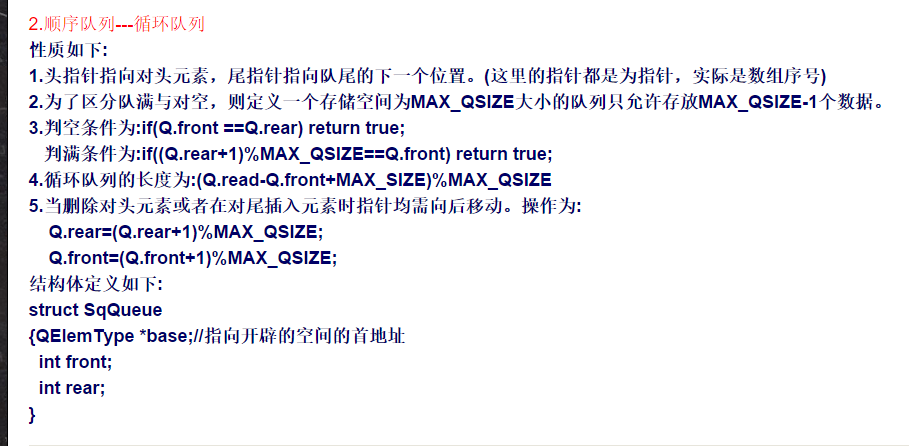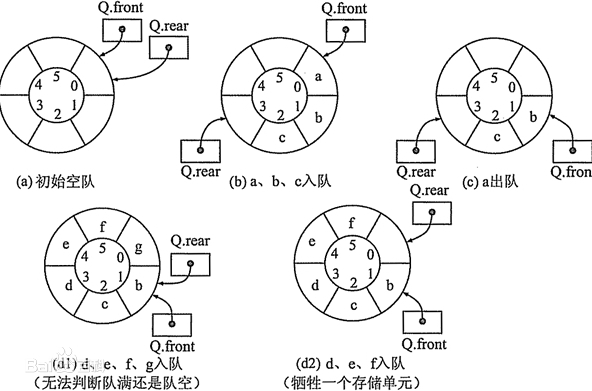• 循环队列的一些基本操作 void InitQueue(SqQueue &Q) { // 构造一个空队列Q Q.base = (QElemType *)malloc(MAX_QSIZE*sizeof(QElemType)); if (!Q.base) // 存储分配失败 exit(OVERFLOW); Q.front = Q....
循环队列的一些基本操作void InitQueue(SqQueue &Q)
{ // 构造一个空队列Q
Q.base = (QElemType *)malloc(MAX_QSIZE*sizeof(QElemType));
if (!Q.base) // 存储分配失败
exit(OVERFLOW);
Q.front = Q.rear = 0;
}

void DestroyQueue(SqQueue &Q)
{ // 销毁队列Q，Q不再存在
if (Q.base)
free(Q.base);
Q.base = NULL;
Q.front = Q.rear = 0;
}

void ClearQueue(SqQueue &Q)
{ // 将Q清为空队列
Q.front = Q.rear = 0;
}

Status QueueEmpty(SqQueue Q)
{ // 若队列Q为空队列，则返回TRUE；否则返回FALSE
if (Q.front == Q.rear) // 队列空的标志
return TRUE;
else
return FALSE;
}

int QueueLength(SqQueue Q)
{ // 返回Q的元素个数，即队列的长度
return(Q.rear - Q.front + MAX_QSIZE) % MAX_QSIZE;
}

Status GetHead(SqQueue Q, QElemType &e)
{ // 若队列不空，则用e返回Q的队头元素，并返回OK；否则返回ERROR
if (Q.front == Q.rear) // 队列空
return ERROR;
e = Q.base[Q.front];//等价于e=*(Q.base+Q.front)
return OK;
}

Status EnQueue(SqQueue &Q, QElemType e)
{ // 插入元素e为Q的新的队尾元素
if ((Q.rear + 1) % MAX_QSIZE == Q.front) // 队列满
return ERROR;
Q.base[Q.rear] = e;//等价于*(Q.base+Q.rear)=e
Q.rear = (Q.rear + 1) % MAX_QSIZE;
return OK;
}

Status DeQueue(SqQueue &Q, QElemType &e)
{ // 若队列不空，则删除Q的队头元素，用e返回其值，并返回OK；否则返回ERROR
if (Q.front == Q.rear) // 队列空
return ERROR;
e = Q.base[Q.front];
Q.front = (Q.front + 1) % MAX_QSIZE;
return OK;
}

void QueueTraverse(SqQueue Q, void(*vi)(QElemType))
{ // 从队头到队尾依次对队列Q中每个元素调用函数vi()
int i;
i = Q.front;
while (i != Q.rear)
{
vi(Q.base[i]);
i = (i + 1) % MAX_QSIZE;
}
printf("\n");
}
详细课件严慧敏数据结构64页
头指针主要用于删除元素的时候，删除时，元素位置向后移，尾指针主要用于在添加元素的时候，添加元素时，尾指针向后移，
一般队列的判空
front == rear
一般队列的判满
为 rear -front ==MAX_QUEUE_SIZE
<STRONG><SPAN style="COLOR: #000066; FONT-SIZE: 18px">void InitQueue(LinkQueue &Q)
{ // 构造一个空队列Q
if(!(Q.front=Q.rear=(QueuePtr)malloc(sizeof(QNode))))
exit(OVERFLOW);
Q.front->next=NULL;
}

void DestroyQueue(LinkQueue &Q)
{ // 销毁队列Q(无论空否均可)
while(Q.front)
{
Q.rear=Q.front->next;
free(Q.front);
Q.front=Q.rear;//释放一块内存要做两点：1.释放指向它的指针。2.将该指针指向空
}
}

void ClearQueue(LinkQueue &Q)
{ // 将Q清为空队列
QueuePtr p,q;
Q.rear=Q.front;
p=Q.front->next;
Q.front->next=NULL;//只留下头结点
while(p)
{
q=p;
p=p->next;
free(q);
}
}

Status QueueEmpty(LinkQueue Q)
{ // 若Q为空队列，则返回TRUE，否则返回FALSE
if(Q.front->next==NULL)//注意不要把链式队列的判空条件与循环队列混淆
return TRUE;
else
return FALSE;
}

int QueueLength(LinkQueue Q)
{ // 求队列的长度
int i=0;
QueuePtr p;
p=Q.front;
while(Q.rear!=p)
{
i++;
p=p->next;
}
return i;
}

Status GetHead(LinkQueue Q,QElemType &e)
{ // 若队列不空，则用e返回Q的队头元素，并返回OK，否则返回ERROR
QueuePtr p;
if(Q.front==Q.rear)
return ERROR;
p=Q.front->next;
e=p->data;
return OK;
}

void EnQueue(LinkQueue &Q,QElemType e)
{ // 插入元素e为Q的新的队尾元素
QueuePtr p;
if(!(p=(QueuePtr)malloc(sizeof(QNode)))) // 存储分配失败
exit(OVERFLOW);
p->data=e;
p->next=NULL;
Q.rear->next=p;
Q.rear=p;
}

Status DeQueue(LinkQueue &Q,QElemType &e)
{ // 若队列不空，删除Q的队头元素，用e返回其值，并返回OK，否则返回ERROR
QueuePtr p;
if(Q.front==Q.rear)
return ERROR;
p=Q.front->next;
e=p->data;
Q.front->next=p->next;
if(Q.rear==p)
Q.rear=Q.front;//如果只有一个节点，那么删除这个节点后rear指针也就丢了，需重新赋值
free(p);
return OK;
}

void QueueTraverse(LinkQueue Q,void(*vi)(QElemType))
{ // 从队头到队尾依次对队列Q中每个元素调用函数vi()
QueuePtr p;
p=Q.front->next;
while(p)
{
vi(p->data);
p=p->next;
}
printf("\n");
}</SPAN></STRONG>
[cpp] view plaincopyprint?
<strong><span style="font-size:18px;color:#000066;">void InitQueue(LinkQueue &Q)
{ // 构造一个空队列Q
if(!(Q.front=Q.rear=(QueuePtr)malloc(sizeof(QNode))))
exit(OVERFLOW);
Q.front->next=NULL;
}

void DestroyQueue(LinkQueue &Q)
{ // 销毁队列Q(无论空否均可)
while(Q.front)
{
Q.rear=Q.front->next;
free(Q.front);
Q.front=Q.rear;//释放一块内存要做两点：1.释放指向它的指针。2.将该指针指向空
}
}

void ClearQueue(LinkQueue &Q)
{ // 将Q清为空队列
QueuePtr p,q;
Q.rear=Q.front;
p=Q.front->next;
Q.front->next=NULL;//只留下头结点
while(p)
{
q=p;
p=p->next;
free(q);
}
}

Status QueueEmpty(LinkQueue Q)
{ // 若Q为空队列，则返回TRUE，否则返回FALSE
if(Q.front->next==NULL)//注意不要把链式队列的判空条件与循环队列混淆
return TRUE;
else
return FALSE;
}

int QueueLength(LinkQueue Q)
{ // 求队列的长度
int i=0;
QueuePtr p;
p=Q.front;
while(Q.rear!=p)
{
i++;
p=p->next;
}
return i;
}

Status GetHead(LinkQueue Q,QElemType &e)
{ // 若队列不空，则用e返回Q的队头元素，并返回OK，否则返回ERROR
QueuePtr p;
if(Q.front==Q.rear)
return ERROR;
p=Q.front->next;
e=p->data;
return OK;
}

void EnQueue(LinkQueue &Q,QElemType e)
{ // 插入元素e为Q的新的队尾元素
QueuePtr p;
if(!(p=(QueuePtr)malloc(sizeof(QNode)))) // 存储分配失败
exit(OVERFLOW);
p->data=e;
p->next=NULL;
Q.rear->next=p;
Q.rear=p;
}

Status DeQueue(LinkQueue &Q,QElemType &e)
{ // 若队列不空，删除Q的队头元素，用e返回其值，并返回OK，否则返回ERROR
QueuePtr p;
if(Q.front==Q.rear)
return ERROR;
p=Q.front->next;
e=p->data;
Q.front->next=p->next;
if(Q.rear==p)
Q.rear=Q.front;//如果只有一个节点，那么删除这个节点后rear指针也就丢了，需重新赋值
free(p);
return OK;
}

void QueueTraverse(LinkQueue Q,void(*vi)(QElemType))
{ // 从队头到队尾依次对队列Q中每个元素调用函数vi()
QueuePtr p;
p=Q.front->next;
while(p)
{
vi(p->data);
p=p->next;
}
printf("\n");
}</span></strong>

详细可见
http://blog.csdn.net/kkkkkxiaofei/article/details/8482066这个博客

展开全文• 一、说明 循环队列就是将队列存储空间的最后一个位置绕到第一个位置，形成逻辑上的环状...因此，队列判空的条件是front=rear，而队列判满的条件是front=（rear+1)%MaxSize。 二、代码 #ifndef CYCLEQUEUE_H #define C
一、说明
循环队列就是将队列存储空间的最后一个位置绕到第一个位置，形成逻辑上的环状空间，供队列循环使用。在循环队列中，当队列为空时，有front=rear，而当所有队列空间全占满时，也有front=rear。为了区别这两种情况，规定循环队列最多只能有MaxSize-1个队列元素，当循环队列中只剩下一个空存储单元时，队列就已经满了。因此，队列判空的条件是front=rear，而队列判满的条件是front=（rear+1)%MaxSize。二、代码
#ifndef CYCLEQUEUE_H
#define CYCLEQUEUE_H
#include <iostream>
#include <vector>
#define MAXSIZE 6 //最大队列长度
using namespace std;
class cycleQueue
{
public:
cycleQueue();
int length();
bool insert(int x);
bool del();
void test();
void print();
private:
int* m_pBase;
int m_front;//头指针，若队列不空，指向队列头元素
int m_rear;//尾指针，若队列不空，指向队列尾元素的下一个位置
};

#endif // CYCLEQUEUE_H


#include "cyclequeue.h"

cycleQueue::cycleQueue()
:m_pBase(nullptr)
,m_front(0)
,m_rear(0)
{
m_pBase = (int*) malloc (MAXSIZE*sizeof(int));
if(!m_pBase){
cout<<"malloc fail"<<endl;
}
}

int cycleQueue::length()
{
return (m_rear - m_front + MAXSIZE) % MAXSIZE;
}

bool cycleQueue::insert(int x)
{
if((m_rear+1)%MAXSIZE == m_front){
cout<<"queue is full"<<endl;
return false;
}
m_pBase[m_rear] = x;
m_rear = (m_rear+1) % MAXSIZE;
return true;
}

bool cycleQueue::del()
{
if(m_front == m_rear){
cout<<"queue is empty"<<endl;
return false;
}
m_front = (m_front+1) % MAXSIZE;
return true;
}

void cycleQueue::test()
{
for(int i=0;i<5;i++){
insert(i);
}
print();
del();
del();
print();
insert(3);
insert(4);
insert(5);
insert(6);
print();
}
void cycleQueue::print()
{
for(int i=0;i<MAXSIZE-1;i++){
cout<<"m_pBase["<<i<<"]:"<<m_pBase[i]<<endl;
}
cout<<"m_front:"<<m_front<<endl;
cout<<"m_rear:"<<m_rear<<endl;
}




展开全文• 数据结构之队列 1.队列介绍 队列： 队列是一种特殊的线性表，特殊之处在于它只允许在表的前端（front）进行...因此，队列判空的条件时front=rear，而队列判满的条件front=（rear+1）%MaxSize。 2.队列与环形队列的比
数据结构之队列
1.队列介绍
队列：
队列是一种特殊的线性表，特殊之处在于它只允许在表的前端（front）进行删除操作，而在表的后端（rear）进行插入操作，队列是一种操作受限制的线性表。进行插入操作的端称为队尾，进行删除操作的端称为队头。队列遵循的原则是先进先出。
环形序列：
环型队列最多只能MaxSize-1个队列元素，当循环队列中只剩下一个空存储单元时，队列就已经满了。因此，队列判空的条件时front=rear，而队列判满的条件front=（rear+1）%MaxSize。
2.队列与环形队列的比较
队列：
front指向队列的头元素的前一个元素，front的初始值为-1，arr[front+1]就能取出第一个元素。
rear也指向队列的头元素的前一个元素，初始值也为-1.
判断队列为空的条件：front==rear
判断队列已满的条件：rear==maxSize-1
环形序列：
front指向队列的第一个元素，front的初始值为0，arr[front]就能取出第一个元素。
rear也指向队列的第一个元素，初始值也为0.
判断队列为空的条件：front==rear
判断队列已满的条件：front==(rear+1)%maxSize
队列中有效数据的个数：(rear+maxSize-front)%maxSize
3.相关代码实现
队列相关代码：
//使用数组模拟一个队列
class ArrayQueue {
private int maxSize;//数组最大容量
private int front;//队列头
private int rear;//队列尾
private int[] arr;//数组用于存放数据

//创建构造器
public ArrayQueue(int maxSize) {
this.maxSize = maxSize;
arr = new int[maxSize];
front = -1;
rear = -1;//指向队列尾
}

//判断队列是否已满
public boolean isFull() {
return rear == maxSize - 1;
}

//判断队列是否为空
public boolean isEmpty() {
return rear == front;
}

//添加数据到队列
public void add(int n) {
if (isFull()) {
System.out.println("队列已满");
return;
}
rear++;
arr[rear] = n;
}

//数据出队列
public int getQueue() {
if (isEmpty()) {
throw new RuntimeException("队列为空，不能取数据");
}
front++;
return arr[front];
}
//显示数列所有数据
public void showQueue(){
//遍历
if(isEmpty()){
System.out.println("队列空的，没有数据");
return;
}
for(int i = 0; i < arr.length; i++){
System.out.println(arr[i]);
}

}
//显示队列的头数据
public int headQueue(){
if(isEmpty()){
throw  new RuntimeException("队列为空");

}
return arr[front+1];
}
}

环形队列相关代码：
class ArrayQueue1 {
private int maxSize;//数组最大容量
private int front;//队列头,初试值为0
private int rear;//队列尾初始值为0
private int[] arr;//数组用于存放数据

//创建构造器
public ArrayQueue1(int maxSize) {
this.maxSize = maxSize;
arr = new int[maxSize];
}

//判断队列是否已满
public boolean isFull() {
return (rear+1)%maxSize == front;
}

//判断队列是否为空
public boolean isEmpty() {
return rear == front;
}

//添加数据到队列
public void add(int n) {
if (isFull()) {
System.out.println("队列已满");
return;
}
//直接将数据加入
arr[rear] = n;
rear=(rear+1)%maxSize;
}

//数据出队列
public int getQueue() {
if (isEmpty()) {
throw new RuntimeException("队列为空，不能取数据");
}
//需要分析front是指向队列的第一个元素
//1.先把front值保存到一个变量
//2,。front后移
//3.将临时变量返回
int value=arr[front];
front = (front+1)%maxSize;
return value;
}
//显示数列所有数据
public void showQueue(){
//遍历
if(isEmpty()){
System.out.println("队列空的，没有数据");
return;
}
//从front开始遍历
for(int i = front; i < front+size();i++){
System.out.println();
}

}
public int size(){
return (rear+maxSize-front)%maxSize;
}
//显示队列的头数据
public int headQueue(){
if(isEmpty()){
throw  new RuntimeException("队列为空");

}
return arr[front];
}
}



展开全文数据结构
• //运行环境keil c51 //学习目的：循环队列的运用 /*关键算法： 在循环队列中，当队列为空...列判空的条件时front=rear，而队列判满的条件时front=（rear+1）%MaxSize。 */ #include "reg51.h" #include <stdio.h>.
//运行环境keil c51
//学习目的：循环队列的运用
/*关键算法：
在循环队列中，当队列为空时，有front=rear，而当所有队列空间全占满时，
也有front=rear。为了区别这两种情况，规定循环队列最多只能有MaxSize-1个
队列元素，当循环队列中只剩下一个空存储单元时，队列就已经满了。因此，队
列判空的条件时front=rear，而队列判满的条件时front=（rear+1）%MaxSize。
*/
#include "reg51.h"
#include <stdio.h>
#include <stdlib.h>
#include <string.h>

#define queue_max  4  //定义最大队列数
typedef struct duilie  //定义队列结构体
{
char buf[queue_max];//定义二维数组，用于存储数据
unsigned char front;
unsigned char rear;
}queue;

queue data_queue;//定义结构体变量
//测试数据定义
char buf={1,2,3};
char read_buf;

//初始化队列，队首，队尾为0
void init_queue(queue *p)
{
if(p==NULL)
return;
p->front =0;
p->rear=0;
}

//判断对列是否满，判断条件：（队尾+1）%队列数 = 队首，则认为对列满；
//这种判断方法有一个弊端，定义最大N个对列，只能存储（N-1）个数据
char queue_full(queue *p)
{
unsigned char temp;
temp = (p->rear+1)%queue_max;
if(temp == p->front)
return 1;
return 0;
}

//判断队列是否为空；
char queue_empty(queue *p)
{
if(p->front == p->rear)
return 1;
return 0;
}

//向队列中写入数据；写入前，判断队列是否满
void queue_push(queue *p,char *p1)
{
if(queue_full(p) == 0)  //队列未满
{
//写入数据
p->buf[p->rear] =  *p1++;
p->buf[p->rear] =  *p1++;
p->buf[p->rear] =  *p1;
p->rear =  (p->rear+1)%queue_max;//更新队尾计数
}
else
return;
}
//从队列中取出数据，取数据前，判断队列是否为空
void queue_pop(queue *p,char *p1)
{
if(queue_empty(p) == 0)//如果为空，不执行取数据操作
{
*p1++ = p->buf[p->front];  //读出当前数据
*p1++ = p->buf[p->front];
*p1   = p->buf[p->front];

p->buf[p->front]=0; //清空存储区
p->buf[p->front]=0;
p->buf[p->front]=0;
p->front =  (p->front+1)%queue_max; //更新头部计数
}
else
return;
}

//主函数
void main()
{
init_queue(&data_queue); //初始化队列
queue_push(&data_queue, buf);//队列函数测试
queue_push(&data_queue, buf);
queue_push(&data_queue, buf);
queue_push(&data_queue, buf);
queue_pop(&data_queue,read_buf);
queue_push(&data_queue, buf);
queue_push(&data_queue, buf);
queue_pop(&data_queue,read_buf);
queue_pop(&data_queue,read_buf);
queue_pop(&data_queue,read_buf);
queue_pop(&data_queue,read_buf);
queue_pop(&data_queue,read_buf);
while (1)
{
P1 = 0x00;
}
}



参考文章链接：https://www.cnblogs.com/TimVerion/p/11194552.html

展开全文• 由于顺序队列有“假溢出”的缺点，所以在应用...循环队列的判读队满的条件是：(Q.rear + 1) % MaxSize == Q.fornt; 下面是队列实现的存储类型结构体： #define MaxSize 50 typedef int ElemType; typedef struct { ...
• 通过该实验，使学生理解链队列的构造特点并灵活应用，掌握链队基本操作的编程实现，认识栈是在一端进行插入，在另一端进行删除集中操作的线性结构，掌握队列的“先入先出”操作特点，知道判断队列空和满的条件，...数据结构 编程语言 c++
• 问题： ...2）画出队列的初始状态，并给出判断队空和队满的条件 3）画出第一个元素入队后的队列状态。 4）给出入队操作和出队操作的基本过程。 解： （1）链式存储 （2） 判空： Q.front = Q.rear 判满数据结构
• ## 队列

2017-09-01 09:50:17
队列的基本操作有初始化队列判队列是否为空，入队，出队 栈可分为两种存储结构：顺序队和链队。 顺序队 /* 顺序队结构 */typedef struct { ElemType data[MAXSIZE]; int front; int rear;} SqQueue; 顺序队四...
• 判满条件为：front=(rear+1)%size
• 循环队列，也叫“环形队列”。 它指的是：将队列抽象成一个环形，如果队头前面有空间，我们...因此，环形队列判空条件是front==rear,判满的条件是(rear+1)%n==front.n表示数组总大小（n=k+1,k表示有效元素个数）...
• 通过该实验，使学生理解链队列的构造特点并灵活应用，掌握链队基本操作的编程实现，认识栈是在一端进行插入，在另一端进行删除集中操作的线性结构，掌握队列的“先入先出”操作特点，知道判断队列空和满的条件，...数据结构 算法 c++
• 通过该实验，使学生理解链队列的构造特点并灵活应用，掌握链队基本操作的编程实现，认识栈是在一端进行插入，在另一端进行删除集中操作的线性结构，掌握队列的“先入先出”操作特点，知道判断队列空和满的条件，...
• 数组模拟队列1.顺序队列2.循环队列 1.顺序队列 指针位置 front：指向队列的第一个元素，也就是说front就是 数组第一个元素。...队列满判断条件if (rear == maxSize - 1) { return true; } 有效队列长度size =java
• 判空和判满的条件都是：q->rear == q->front。带来的问题就是当出现上述条件时不能区分循环队列到底是空还是满，因此为了解决上述问题。人们提出以下两种方案来解决： （1）牺牲一个位置用作判断的条件 队...
• 对于一个无界阻塞队列而言，其实现非常简单，即用一个锁（锁队列）+ 一个条件变量（空）即可。那么对于一个有界阻塞队列而言，其队列的容量有上限，其实只要再加一个条件变量用来判断是否即可。 综上，我们...c++
• 第三章 栈和队列;学习提要 1....熟练掌握循环队列和链队列的基本操作实现 算法特别注意队满和队空描述方法 重难点内容 顺序栈相关操作循环队列的判空判满;3.1 栈stack;栈定义和特点 定义限定仅
• 循环队列的判空，判满条件要综合考虑（可以推导出来） 遍历时候，需要是由到当前size，然后通过取模操作取出循环队列值 code package com.wrial.queue; /* * @Author Wrial * @Date Created in 15:40 ...
• 通过该实验，使学生理解链队列的构造特点并灵活应用，掌握链队基本操作的编程实现，认识栈是在一端进行插入，在另一端进行删除集中操作的线性结构，掌握队列的“先入先出”操作特点，知道判断队列空和满的条件，...数据结构 算法 C
• 1.队列的判空判满条件以及当插入和删除元素时各种可能性； 　比如last元素在末位置，而first元素并不在初始位置； 　又比如，last 元素不在末位置，first元素也不再初始位置等等。 2.队列的数组元素 1 ...
• 通过该实验，使学生理解链队列的构造特点并灵活应用，掌握链队基本操作的编程实现，认识栈是在一端进行插入，在另一端进行删除集中操作的线性结构，掌握队列的“先入先出”操作特点，知道判断队列空和满的条件，...
• 基本思路：栈具有先进后出特性，所以我们用s1来存储进队元素，用s2来出队，在这个过程中，由于s1出栈数据是逆序，再压入s2再次逆序，那么从s2输出数据就是正常顺序，用这样思路模拟一个队列。...数据结构
• 文章目录一、写在前言二、队列（一）队列介绍（二）数组模拟队列（三）循环队列1、判满条件2、队列元素个数（四）循环队列代码实现三、结束语 一、写在前言 大二下在学校学习数据结构，老师虽然长很漂亮又温柔，...数据结构 算法 java
• 1. 初始化 数组 头尾节点 2. 插入 3. 判满 ...需要预留一个位置，用于判断队列是否满了 ...判断队列的条件： rear==front; 打印整个队列： for循环所需条件： 开始下标：front 有效个数：(rear+maxSize-数据结构 算法